# SQL Aggregate Window Functions With Examples & Practice Exercises

Previously in the intermediate SQL tutorial, you learned about aggregate functions like , , , , and . Now, let's take these commands a step further by performing aggregations over specific window(s) or subset of rows using a window function.

#### Introduction to Window Functions

What exactly are window functions?

In simple terms, they are functions that operate by creating virtual "windows" 🪟 within the dataset.

To demonstrate this, let's analyze the dataset from this Amazon SQL interview question, which contains information on products purchased by Amazon customers.

In our dataset below, we can make 7 of these virtual "windows" 🪟 – one for each .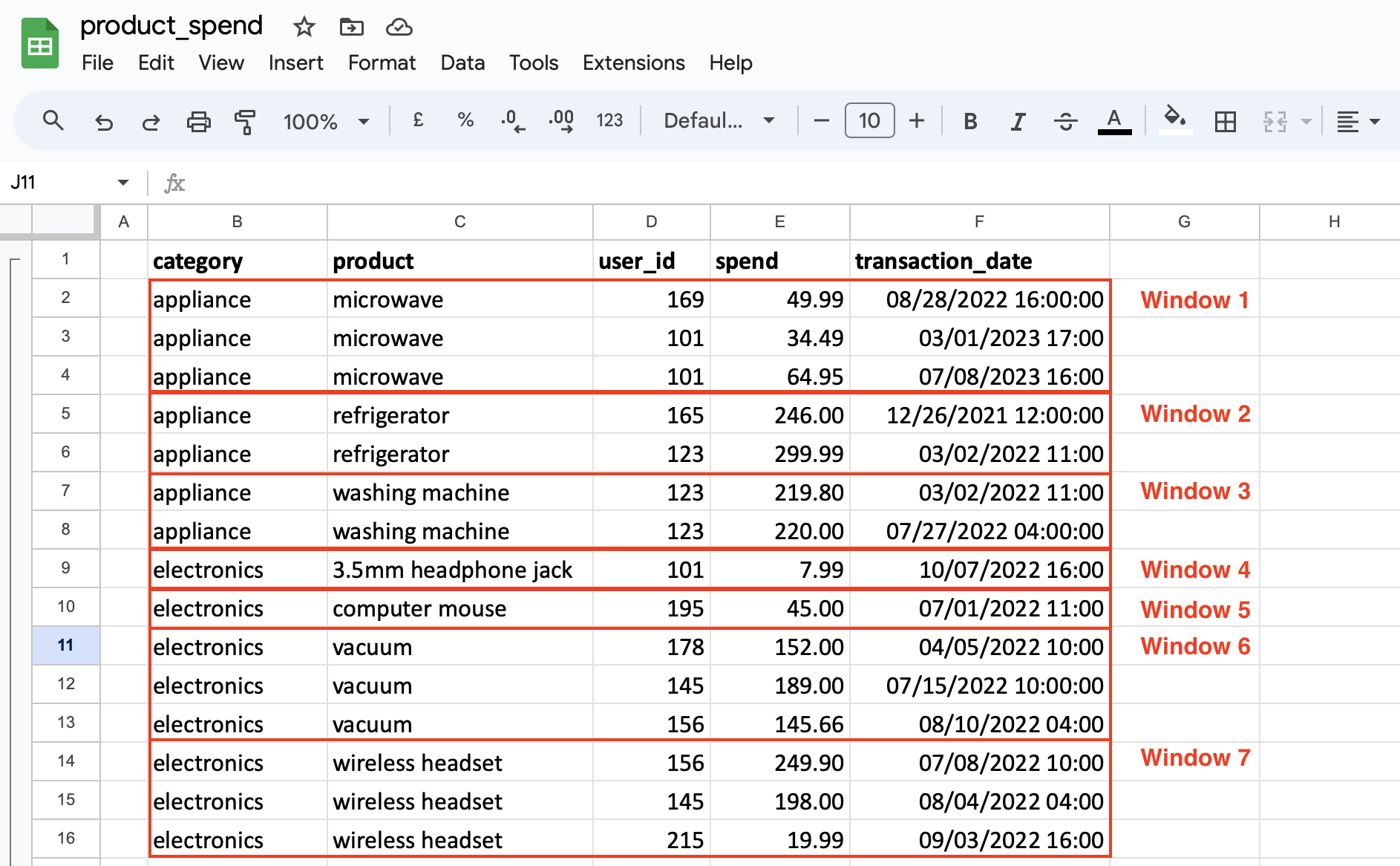Each window operates independently, so we can do aggregate functions like or just on a window.

Take a look at this example, which creates a running total of spend for each product type:

You can see that the above query sums up for each window. We have the results in the image below: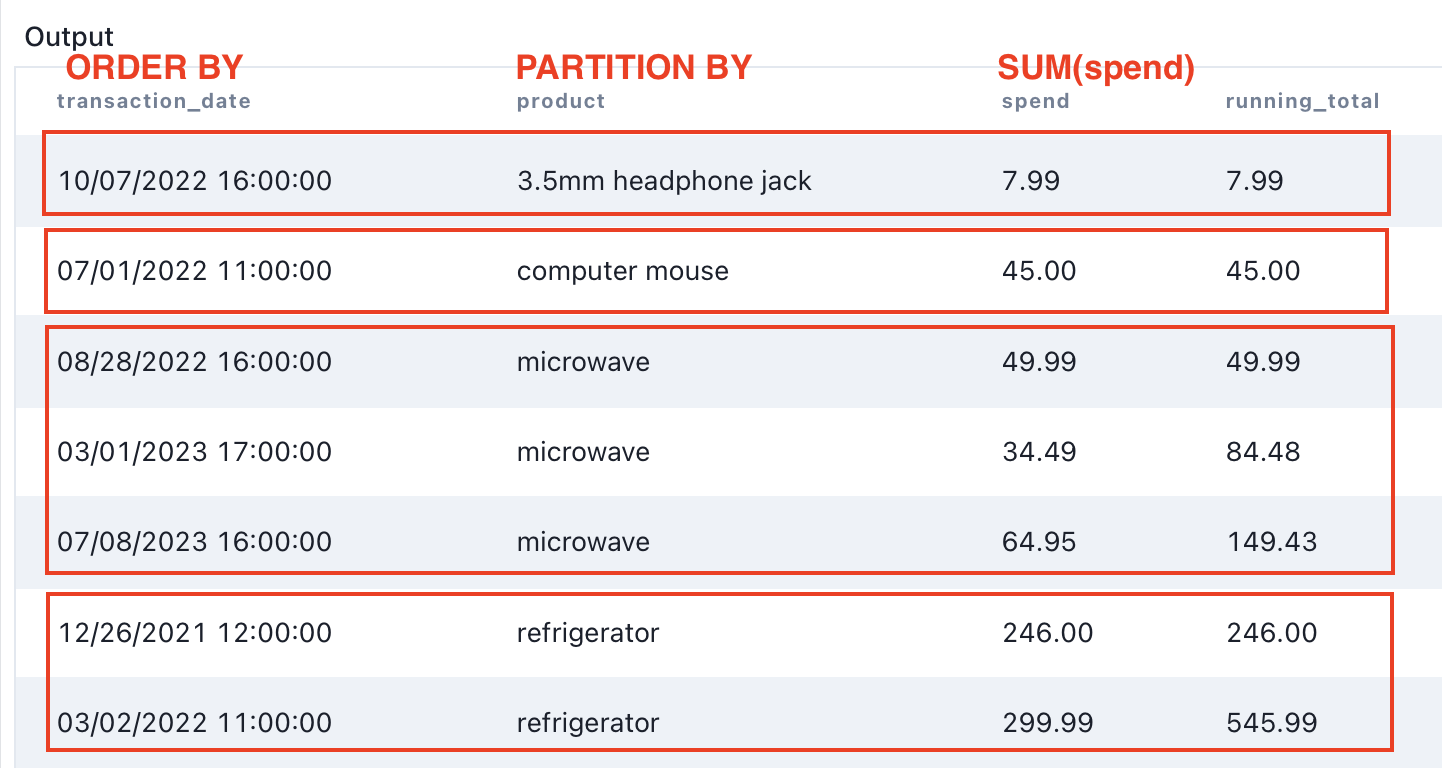We're calculating the sum of spending for the whole dataset, but we're organizing it into sections/partitions/windows by . Within each section/window, we arrange/order the data by , and we're adding up spending as we go down the section.

Notice how the starts over when we switch to the next window!

#### Breaking Down The Window Function

Let's break down the earlier window function:

Here's what each SQL command in the window function is doing:

• SUM(): is a typical aggregate function
• OVER: required for window functions
• PARTITION BY: makes each it's own section / window,
• ORDER BY: the data is ordered by , and the accumulates the sum across the current row and all subsequent rows of
• ORDER BY: essentially sorts the data by the specified column, similar to an clause.
• Without , each value would be a sum of all the values without its respective .

Try running the below query without to see the difference:

Now that we've established the foundation for window functions, we'll dive deeper into the 7 most frequently used aggregate window functions.

### Common Aggregate Window Functions

In this tutorial, we'll explore the 7 commonly used SQL Aggregate Window Functions:

• counts the number of rows in a specified column across a defined window.
• computes the sum of values within a specified column across a defined window.
• calculates the average of a selected group of values across a defined window.
• retrieves the lowest value from a particular column across a defined window.
• fetches the highest value from a specific column across a defined window.
• returns the first value in a designated column across a defined window.
• provides the last value in a given column across a defined window.

#### COUNT(): Count Rows Within a Window

It's pretty self-explanatory, but in case you're new to aggregate window functions, we'll guide you through.

### Querying without Partition

In this query, we did not specify any columns for partitioning, hence we're counting the number of rows within the entire dataset.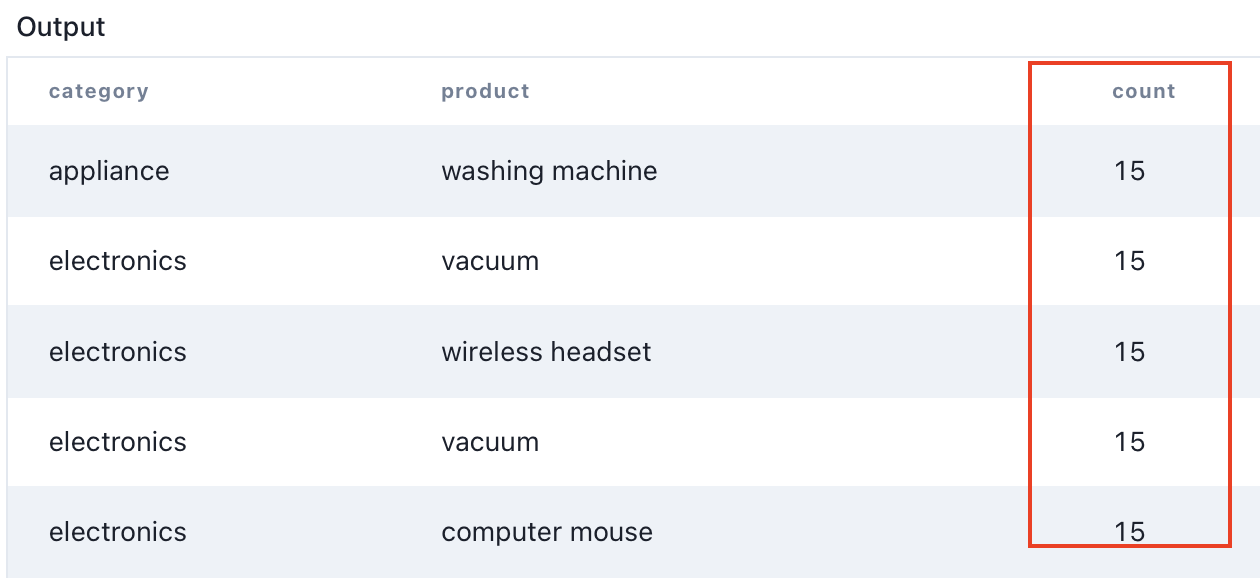#### Partitioning with a Single Column: Category

In this query, we're partitioning the results by column:

Upon executing this query, you'll notice that the category count of appliances is 7, and for electronics, it's 8. This time we're counting the number of rows within the partition. Are you seeing a pattern now?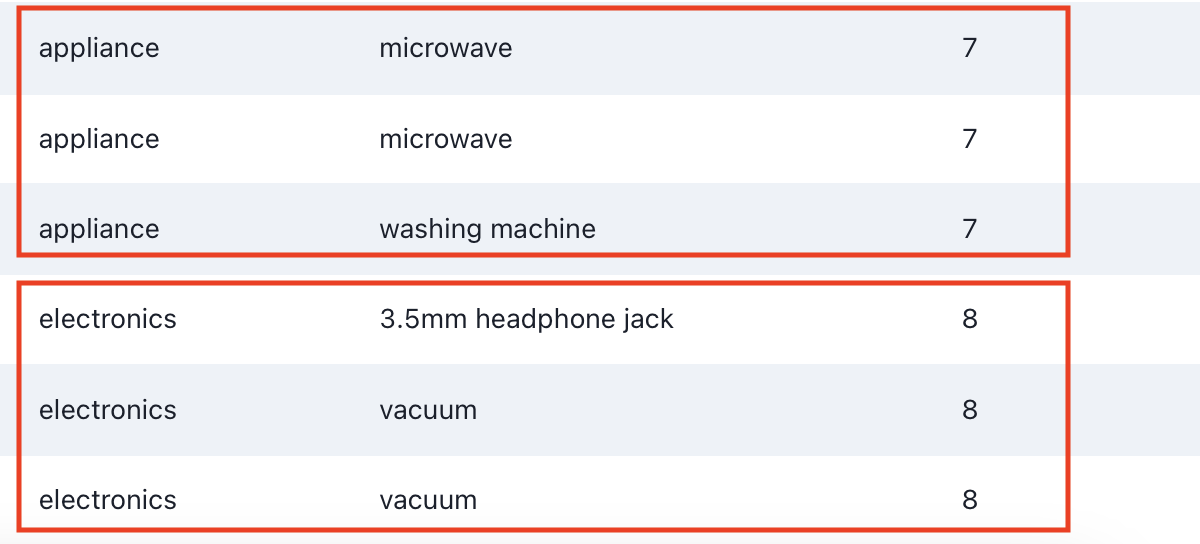#### Partitioning with Two Columns: Category and Product

In this query, we're partitioning the results by and columns:

Now, you'll notice that the function is counting the number of rows within the and column.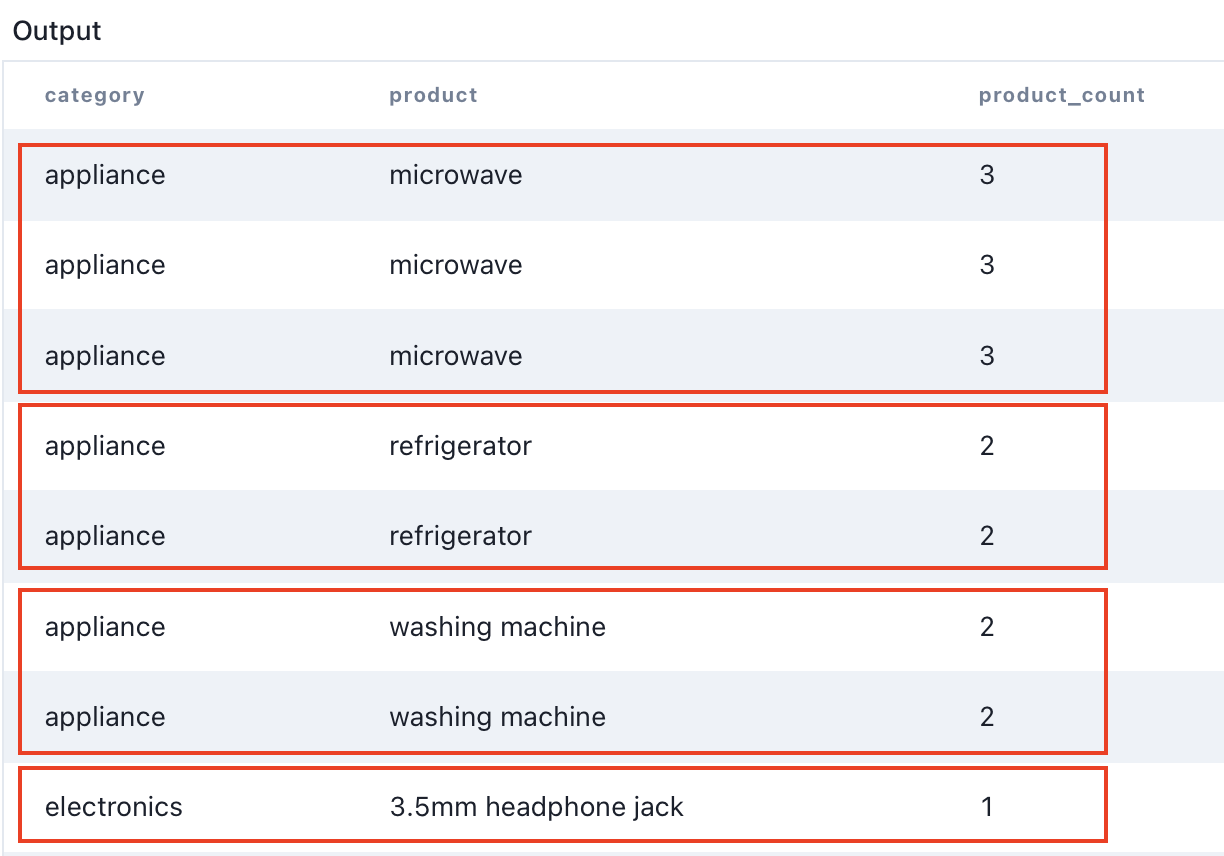#### SUM(): Calculating Running Sum Within a Window

The window function calculates the running or cumulative sum of values within a specified column across a defined window.

In this query:

• The clause divides the data into partitions based on .
• calculates the cumulative sum of within each partition.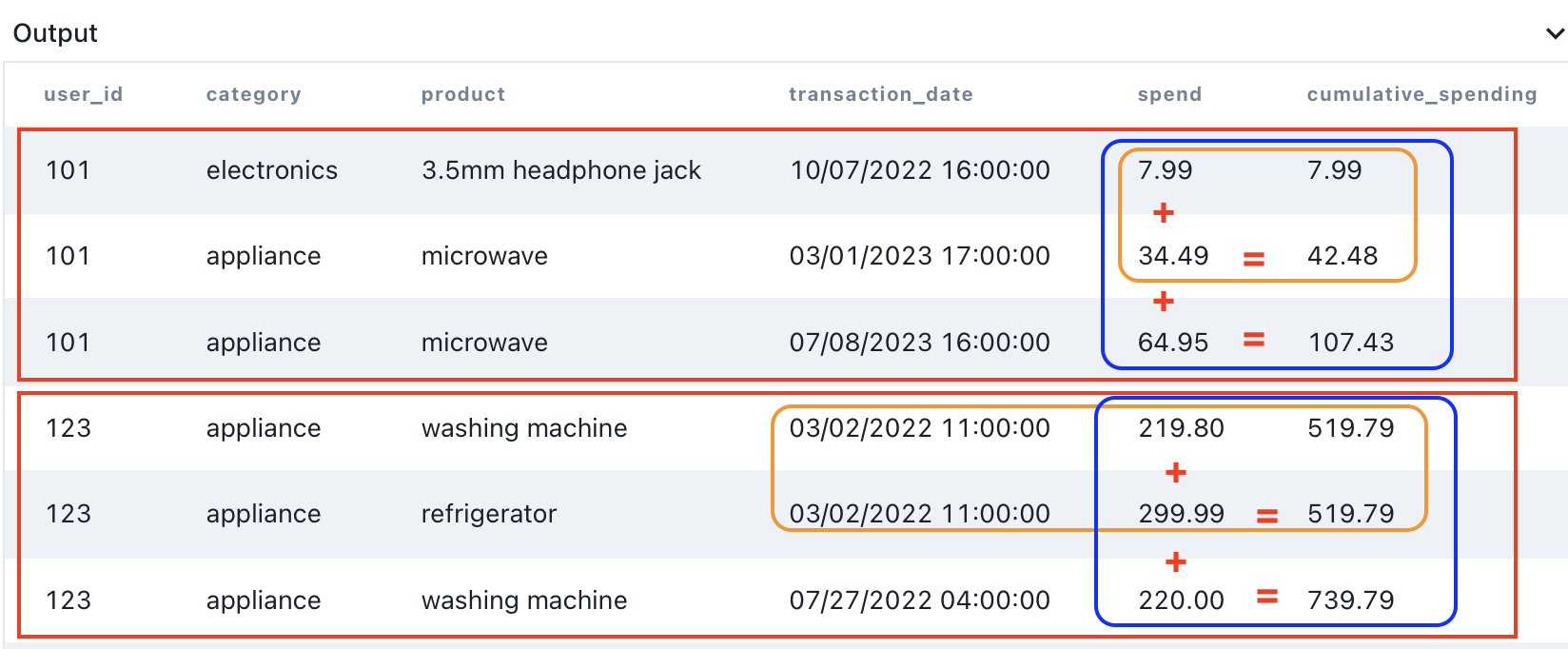Let's break down the results together:

First window for user 101:

1. In the first row, the spend is \$7.99. Therefore, the cumulative spending remains \$7.99.
2. Moving on to the next row, the cumulative spend becomes \$7.99 + \$34.49 = \$42.48. And then, with the subsequent row, it becomes \$7.99 + \$34.49 + \$64.95= \$107.43.

Second window for user 123:

1. Here's where the scenario is slightly different. The cumulative spend becomes \$219.80 + \$299.99 = \$519.79, as both products were purchased at the same time.
2. As we progress to the next row, the cumulative spending continues to accumulate: \$219.80 + \$299.99 + \$220.00 = \$739.79.

## AVG(): Calculate Rolling Averages Within a Window

The window function computes rolling averages of selected values within a specified column.

In this query:

• We determine the average of values for each partition formed by .
• calculates the average within each partition.
• The function rounds the average to 2 decimal places.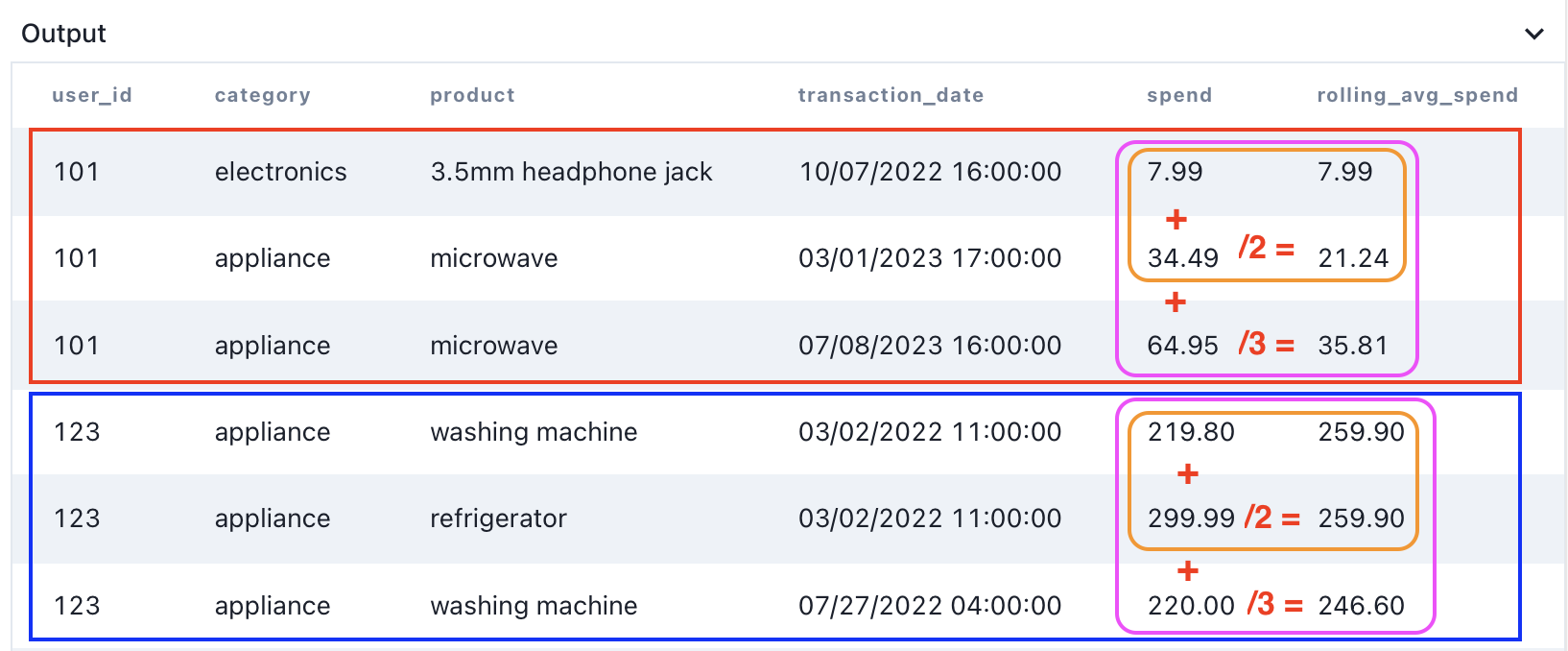Let's break down the results together:

First window for user 101:

1. In the first row, the spend is \$7.99. Therefore, the rolling average spend remains \$7.99.
2. In the next row, the rolling average becomes (\$7.99 + \$34.49)/2 = \$21.24. And then, in the following row, it becomes (\$7.99 + \$34.49 + \$64.95)/3 = \$35.81.

Second window for user 123:

1. This is slightly different. The rolling average becomes (\$219.80 + \$299.99)/2 = \$259.90, as both products were purchased at the same time.
2. As we progress to the next row, the rolling average continues to accumulate: (\$219.80 + \$299.99 + \$220.00)/3 = \$739.79.

Try out the Twitter SQL question here!

#### MIN(): Returns the Lowest Value Within a Window

The window function returns the lowest value from a specified column over a given window.

In this query:

• We retrieve the minimum spend value within each partition formed by .
• The clause divides the data into these partitions.
• The function then identifies the lowest spend value within each partition.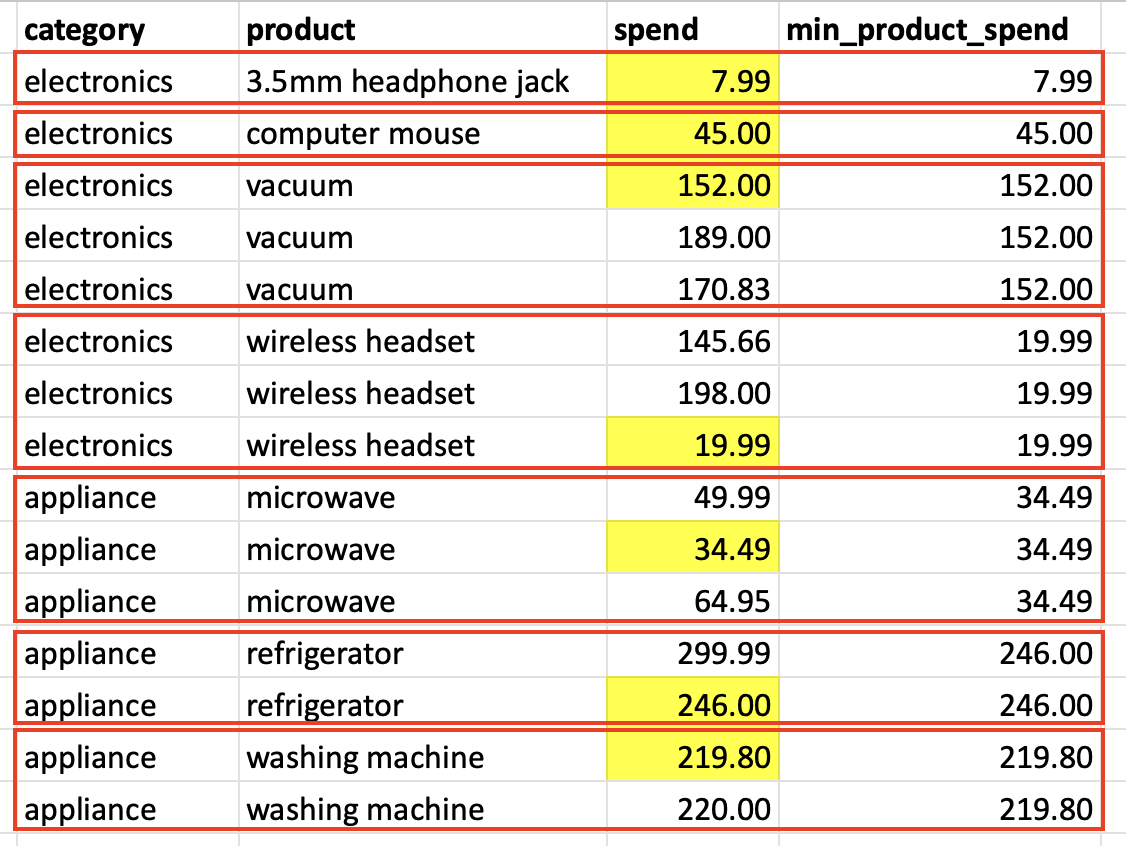#### MAX(): Returns the Highest Value Within a Window

The window function returns the highest value from a specified column over a given window.

In this query:

• We extract the maximum spend value within each partition created by .
• The clause divides the data into these partitions.
• The function then identifies the highest spend value within each partition.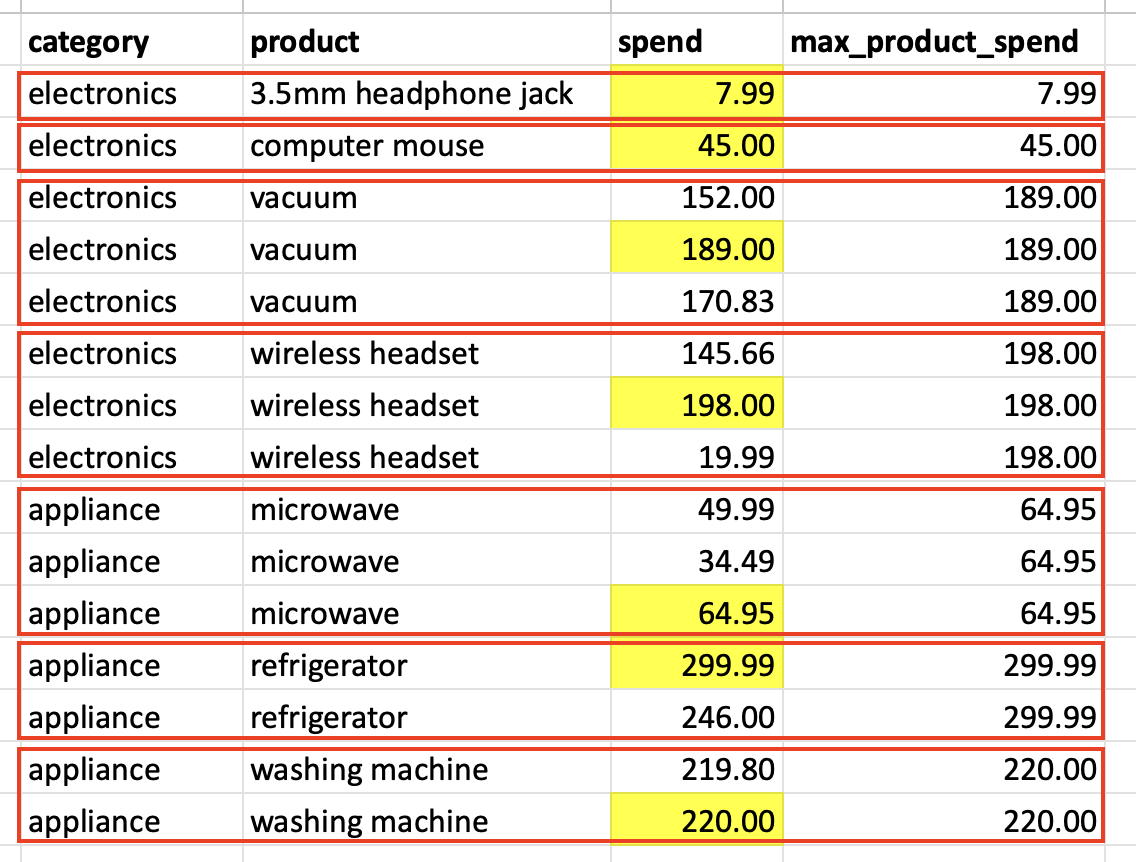#### FIRST_VALUE() and LAST_VALUE(): Returns First and Last Values

The function retrieves the value of a specified column from the first row of a given window. Conversely, the function extracts the value of a specified column from the last row of a given window.

##### FIRST_VALUE() and LAST_VALUE() over the Entire Result Set

The following example uses and to return all products and their first and last purchases made by all users, sorted by transaction date.

In this query:

• The functions treated the whole result set as a single partition.
• The clause arranges products by `transaction_date, from the oldest to the most recent.
• extracts the first product value, and extracts the last product value from the result set.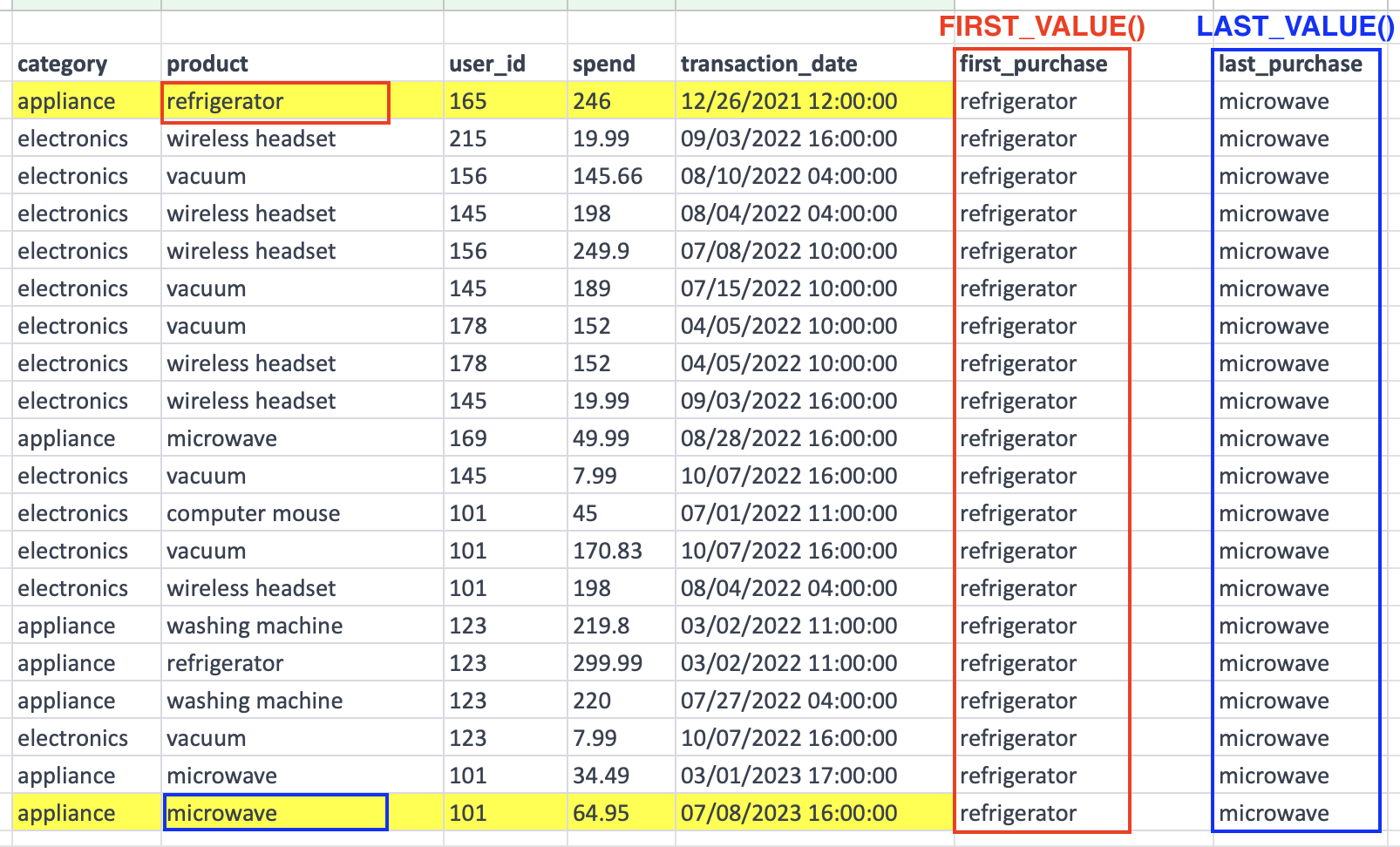##### FIRST_VALUE() and LAST_VALUE() within Partitions

The query is similar to the previous one, but it returns the first and last purchases made by each user.

In this query:

• The clause groups by user.
• The clause sorts by within each partition.
• extracts the first product value, and extracts the last product value for each user.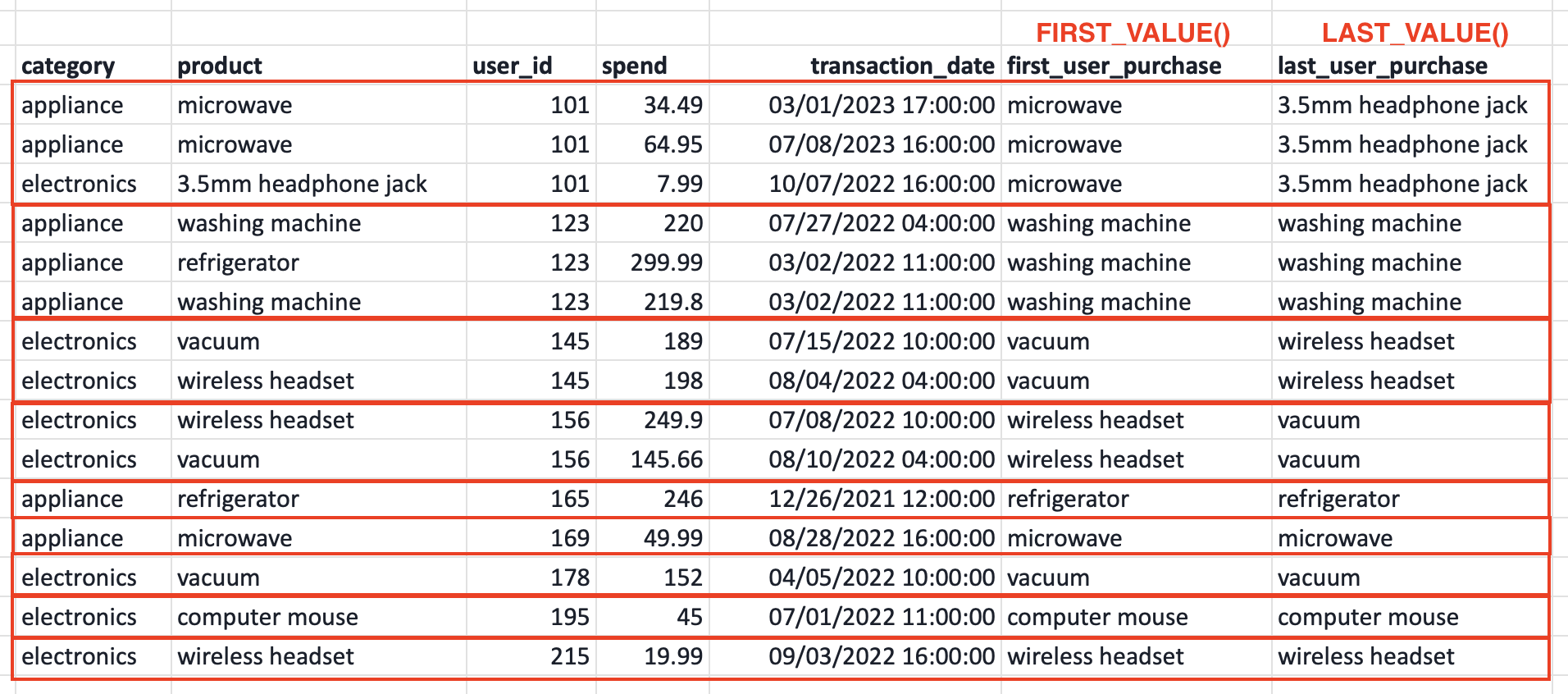### JP Morgan Aggregate Window Function SQL Interview Question

Try this JPMorgan SQL question here to practice using aggregate window functions!

Next Lesson

SQL RANKING 🥇